## RS Aggarwal Class 7 Solutions Chapter 2 Fractions Ex 2B

These Solutions are part of RS Aggarwal Solutions Class 7. Here we have given RS Aggarwal Solutions Class 7 Chapter 2 Fractions Ex 2B.

Other Exercises

Question 1.
Solution: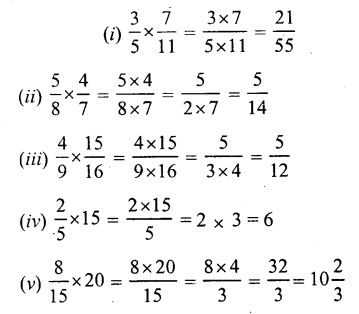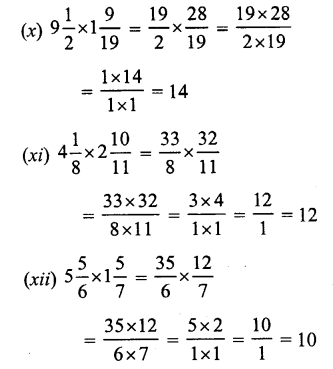Question 2.
Solution: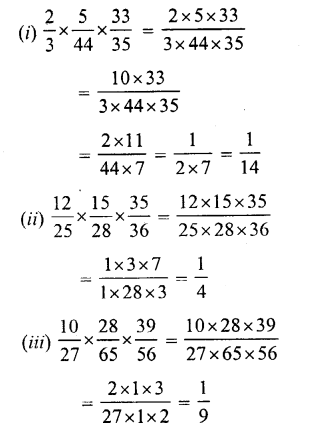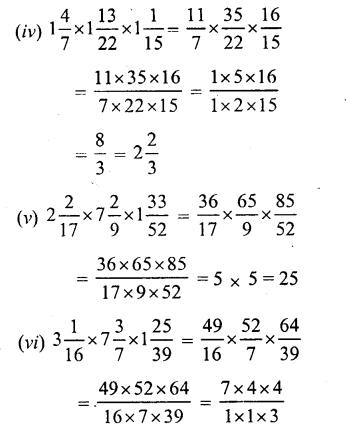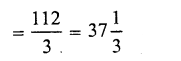Question 3.
Solution: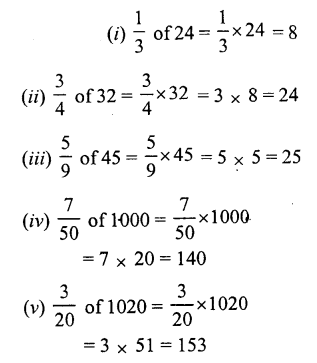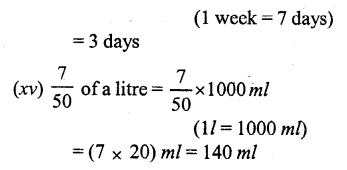Question 4.
Solution:Question 5.
Solution:
Cost of 1 metre pf cloth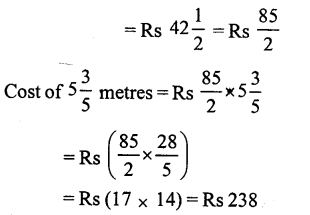Question 6.
Solution:
Distance covered in 1 hourQuestion 7.
Solution: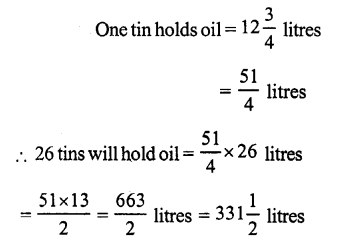Question 8.
Solution: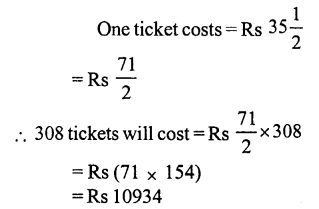Question 9.
Solution: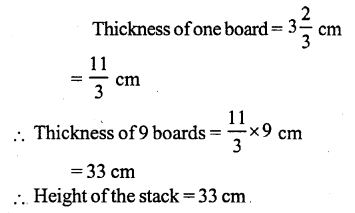Question 10.
Solution: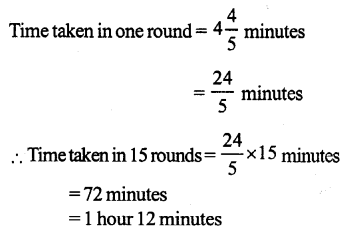Question 11.
Solution:
Weight of Amit = 35 kg.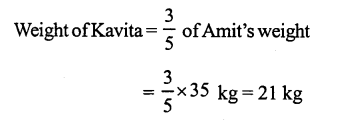Question 12.
Solution:
Total number of students in a class = 42
Number of boys = $$\frac { 5 }{ 7 }$$ of 42 = 5 x 6 = 30
Number of girls = 42 – 30 = 12

Question 13.
Solution:
Sapna total income for one month = Rs 24000
Amount spent = $$\frac { 7 }{ 8 }$$ of her income
= $$\frac { 7 }{ 8 }$$ x 24000
= Rs (7 x 3000) = Rs 21000
Amount deposited in the bank per month = Rs 24000 – 21000 = Rs 3000

Question 14.
Solution:
Length of each side of a square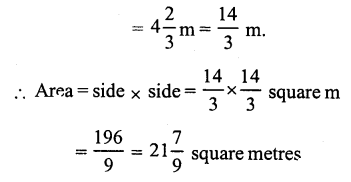Question 15.
Solution:
Length of rectangular field (l)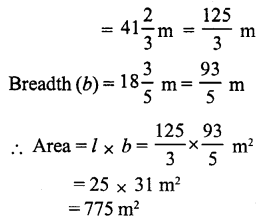Hope given RS Aggarwal Solutions Class 7 Chapter 2 Fractions Ex 2B are helpful to complete your math homework.

If you have any doubts, please comment below. Learn Insta try to provide online math tutoring for you.